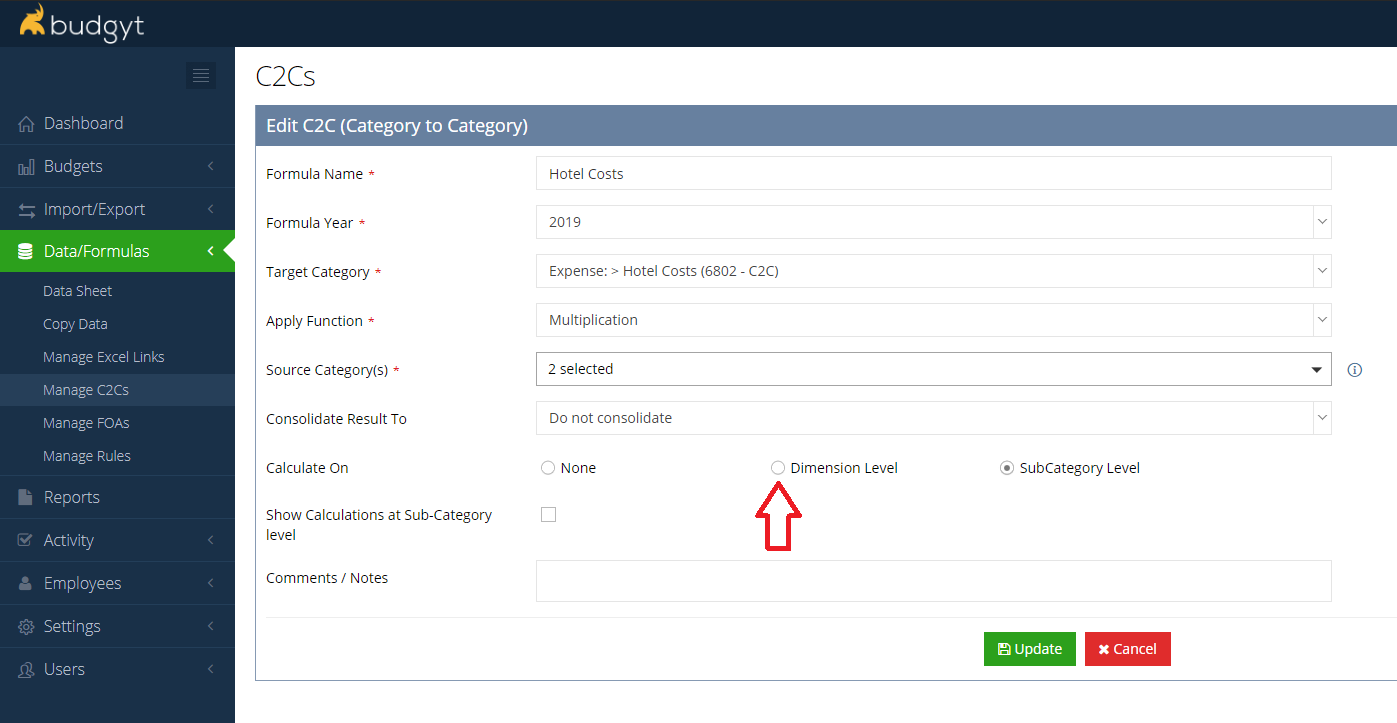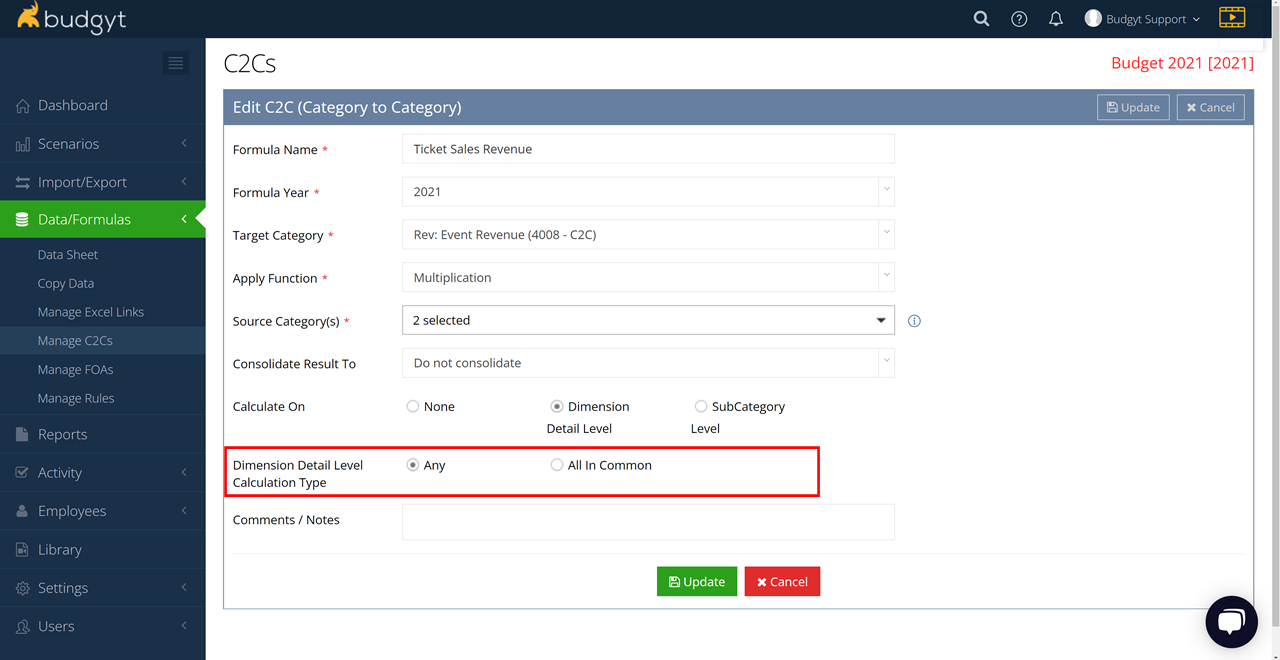# C2C Formulas using Dimensions

## Calculate results for each Dimension in your inputs

The standard operation of the C2C formula calculates the result of your formula by either working with the total of each source category, or summing up the totals at the sub category line level (for instance if you click the "Calculate at Sub Category" option, you might multiple sub category 1 in source category A x sub category 1 in source category B, line 2 x line 2, line 3 x line 3 etc).

With Dimensions switched on you will see an additional option in your C2C edit screen - Dimensions Level.This will match up Dimensions in your source categories, so you will only get a result when you have the same dimension in each of your source categories. Like Dimensions do not need to appear on the same row for the formula to work.

See table below for an example:You can also choose how the Dimensions are used in the calculation by checking the Any in Common, or All in Common buttons:If you choose All in Common, the formula will only work if each of the inputs has the exact same dimensions selected, e.g. Source Category A has an entry with Dimensions 1, 2, and 3 selected, and Source Category B has an entry that also has Dimensions 1, 2, and 3 selected. If one is missing, there will be no calculation.

If you choose Any in Common, there only needs to be one common dimension in each of the inputs, and you will get a result. This is particularly useful if you have a formula where one of the inputs will always be the same no matter which dimension is selected, e.g. if you use calendar days as an input.